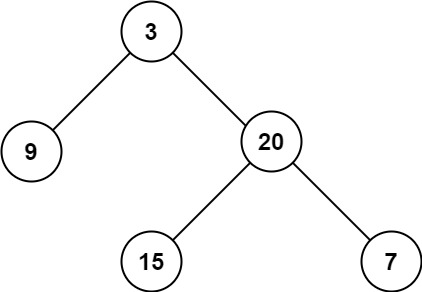# 104. Maximum Depth of Binary Tree / Easy

Given the root of a binary tree, return its maximum depth.

A binary tree’s maximum depth is the number of nodes along the longest path from the root node down to the farthest leaf node.

## Example 1:Input: root = [3,9,20,null,null,15,7]
Output: 3

## Example 2:

Input: root = [1,null,2]
Output: 2

## Constraints:

• The number of nodes in the tree is in the range [0, 10^4].
• -100 <= Node.val <= 100

# Solution: Recursive

## 效能

### Complexity

• Time Complexity: O(N)
• Space Complexity: O(N), best case is O(logN)

### LeetCode Result

• Runtime: 13 ms
• Memory Usage: 18.8 MB